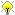[Documentation] [TitleIndex] [WordIndex]

 Note: This tutorial assumes that you have completed the previous tutorials: Capturing data from a controller.Please ask about problems and questions regarding this tutorial on answers.ros.org. Don't forget to include in your question the link to this page, the versions of your OS & ROS, and also add appropriate tags.

# Plotting controller data in matlab or octave

Description: This tutorial teaches you how to visualize the captured state of a controller in matlab or octave.

Tutorial Level: INTERMEDIATE

## Introduction

We have previously seen an example of how to capture data from a controller. This data, however, is usually only useful if it can be visualized, analyzed, or otherwise processed. In this tutorial, we load the data into matlab for easy plotting and examination. Wherever and whenever we refer to matlab, octave can be used in place.

## Saving the data to file

As a first step, we save the data published from the controller into a file. This is best achieved via rostopic using the 'echo -p' command to format the data in matlab friendly form.

With the controller running (see the tutorial on capturing data from a controller), listen to the topic with redirection into a file:

`  \$ rostopic echo -p /my_controller_name/mystate_topic > datafile.rtp`

Notice we use a 'rtp' extension to denote the rostopic, but any other extension would be equally valid. To capture the data, trigger the service from a separate prompt:

`  \$ rosservice call /my_controller_name/capture`

If you are only interested in a portion of the data, for example one second, you may wish to pass through the unix 'head' command to limit the number of lines:

`  \$ rostopic echo -p /my_controller_name/mystate_topic | head -n 1001 > datafile.rtp`

This will not only limit the size of the data, but also terminate the 'rostopic' command when completed.

If you examine the data file datafile.rtp, you will notice a single header line. This initial line describes the data format and is very useful when loading into matlab. In our example, the header line will look like

`%time,field.dt,field.position,...`

This is why the 'head' command stored 1001 lines, to include the header and 1000 samples.

Note, this code only works for Matlab <2009b.

Loading the data into matlab can be very efficient/powerful, if we allow matlab to use the header line to automatically name the data. To do this, create the file rtpload.m somewhere in your matlab path, for example in current directory. Copy and paste the following function definition:

```   1 function  [time, data] = rtpload(filename)
2 %
3 %   [time, data] = rtpload(filename)
4 %
5 %   Load data from a ROS message file, created with
6 %   a 'rostopic echo -p topic > filename' command.
7 %
8 %   filename    name (including path) of data file
9 %   time        Nx1 vector of ROS times, when the
10 %                individual messages were received
11 %   data        structure of Nx1 vectors, corresponding
12 %                to the fields in the message data
13
14 % Note: rostopic saves a header line with information
15 % about the data.  The first column is the time the
16 % message was received, the rest are message fields.
17
18 % Get the header line - which includes the data format.
19 fid = fopen(filename);
20 if (fid < 0)
21   error('Unable to open file %s', filename);
22 end
23 line = fgetl(fid);
24 fclose(fid);
25
26 % Make sure the file contains something.
27 if (line <0)
28   error('Empty file %s', filename);
29 end
30
31 % Load the actual data.
33
34 % Restructure the data.
35 column = 0;
36 while (~isempty(line))
37   [token,line] = strtok(line,'%,');
38   column = column+1;
39   eval([token ' = raw(:,' num2str(column) ');']);
40 end
41
42 % Move to the correct output variables.  The first column
43 % is 'time', the rest are 'field.item1' 'field.item2' etc.
44 time = time;
45 data = field;
46
47 return;
```

This function

3. restructures the data according to the header line.

For example, when looking at data from the controller using our previous message definition, we end up with two variables:

1. 'time', which is simply a vector of the ROS times at which the individual messages were received. As our controller publishes all data at once, this time is not very useful.
2. 'data', which is a structure of the fields inside a message. So we will see

```data.dt
data.position
data.desired_position
data.velocity
data.desired_velocity
data.commanded_effort
data.measured_effort```

Using this 'rtpload' function, we can load the previously stored data. Inside matlab execute:

`  >> [time, data] = rtpload('datafile.rtp');`

And then we can plot the signals. For example

`  >> plot([data.position data.desired_position]);`

As the 'time' variable is not very useful when looking at data from a controller, we can reconstruct time using:

```  >> t = cumsum(dt);
>> plot(t, data.position);```

except to date, the 'dt' variable was simply set to zero and hence the 't' vector will also be zero. However, as we start tuning the system, we will use a valid 'dt'.

2020-01-25 12:44